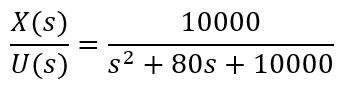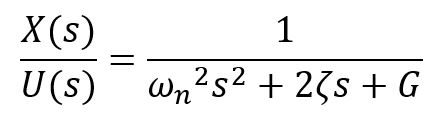Robotics 2
Controller Tuning from a Model
QuizQuestion 3:  What is a 'transfer function' of a system?
Question 4:  Which of these is the standard form of a transfer function of a second-order system?
Question 1:  In controls terminology, what is a 'plant'?
Question 2:  Mathematically, what does a 'Laplace Transform' do?
The system we are trying to control
It converts a differential equation into an algebraic equation
Question 5: When a system is 'critically-damped', what is the value of the damping ratio, zeta?Question 9:  What will be the natural frequency of the system in Question 6 when it is critically damped?  Round to two places after the decimal.
Question 6:  Suppose that I have a system that I am controlling with proportional control, and I have set Kp=1, and have determined that this system is a second-order system that has a damping ratio of 0.4, a natural frequency of 100 rad/s, and a gain of 1.  Which of these equations is the transfer function of the system?
Question 8:  For the system in Question 6, now suppose that I want a damping ratio of 0.7 instead.  What value should I set for Kp?  Round to two places after the decimal.
Question 10:  I can expect that the value of Kp calculated in Question 7 will be only approximate when I apply it to my actual system.  Which of these explains some of the reasons for the discrepancy?
Question 7:  Suppose that I would like the system in Question 6 to have a damping ratio of 1.0.  What value should I set for Kp?  Round to two places after the decimal.
The complete feedback control system
The control equation, like PID
The results of control, like a step response
It converts a feedback system into an open-loop system
It converts a partial differential equation into an ordinary differential equation
It converts an equation in the time domain into an equation in the frequency domain
The output of the system divided by the input
The input of the system divided by the output
The Laplace transform of the system
The frequency response of the systemIn our analytical approach, we can only have a proportional controller (Kp).  But, in reality we might want a more complex controller like PI, PD, or PID.  Our analytical methods won't work with these other controllers.
In our analytical approach, we are assuming that the plant is perfectly linear.  But, the real plant has nonlinearities such as static and dynamic friction.  Also, our analytical controller design methods assume that the control loop operates infinitesly fast.  But, in reality, executing one control loop takes a finite amount of time.
Our analytical methods will only work for first-order or second-order systems.  But, real systems might be third-order or higher.  So, our analytical methods won't work for these systems.